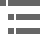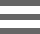## Polarized Light WaveformsThe ordinary and extraordinary light waves generated when a beam of light traverses a birefringent crystal have plane-polarized electric vectors that are mutually perpendicular to each other. In addition, due to differences in electronic interaction that each component experiences during its journey through the crystal, a phase shift usually occurs between the two waves. This interactive tutorial explores the generation of linear, elliptical, and circularly polarized light by a pair of orthogonal light waves (as a function of the relative phase shift between the waves) when the electric field vectors are added together.

The tutorial initializes with two orthogonal sine waves (the electric vector components of the waves are colored red and blue) traveling from left to right in the window, and having a 90-degree (one-quarter wavelength) out-of-phase relationship. Circumscribing the mutually perpendicular waves is a helical black line (resultant vector) that is generated by calculating the sum of the electric vector components from each wave. The relative phase shift between the two waves can be altered with the Phase Shift slider through a range of zero to 360 degrees. As this slider is translated to the left or right, one wave moves in relation to the other along the propagation axis (shifting the phase), and the black line representing the vector sum changes from elliptical to circular or linear, depending upon the value of the phase relationship between the waves. Translation of the sine wave across the tutorial window can be paused at any point by clicking on the Pause button, and the tutorial is re-initialized by clicking on the Reset button. The Speed slider controls the rate of translation by the sine waves across the tutorial window.

In order to view the sine waves from a variety of angles, use the mouse cursor to click and drag the waves anywhere within the boundaries of the tutorial window. The waves both terminate simultaneously in a gray box positioned at one end of the waves. Within the gray box, the undulating electric vector component from each wave is represented by a black square that vibrates in the same plane as the parent wave. In addition, the resultant of the electric vector summation between the two waves is represented by a thick black arrow that moves back and forth in the gray box to scribe either a line, ellipse, or circle, depending upon the phase relationship between the orthogonal waves. The gray box and resultant arrow can be eliminated from the window by removing the checkmark from the Show Resultant check box.

When the Phase Shift slider is set to zero, 180, and 360 degrees, the resultant vector (the black line surrounding the waves or the thick arrow in the gray box) creates a black sine wave positioned at a 45-degree angle between the orthogonal waves, or traces a straight line when the approaching waves are viewed end-on from the gray box. Between zero and 90 degrees, the resultant vector forms an ellipse (representing elliptically polarized light) around the waves and in the gray box. At 90 degrees, the ellipse becomes a circle (representing circularly polarized light). In both cases, the sweep of the resultant vector is counterclockwise, which indicates left-handed polarized light. As the slider is translated between 90 and 180 degrees, the ellipse slowly collapses to form linearly polarized light (at 180 degrees; in the opposite quadrant from the orientation at zero degrees), and then right-handed elliptically polarized light when the slider is moved past 180 degrees. At 270 degrees, right-handed circularly polarized light is produced, which folds into elliptically polarized light between 270 and 360 degrees and, finally, linearly polarized light is again formed at 360 degrees.

In linearly polarized light, the electric vector is vibrating in a plane that is perpendicular to the direction of propagation, as discussed above. Natural light sources, such as sunlight, and artificial sources, including incandescent and fluorescent light, all emit light with orientations of the electric vector that are random in space and time. Light of this type is termed non-polarized. In addition, there exist several states of elliptically polarized light that lie between linear and non-polarized, in which the electric field vector transcribes the shape of an ellipse in all planes perpendicular to the direction of light wave propagation.

Elliptical polarization, unlike plane-polarized and non-polarized light, has a rotational "sense" that refers to the direction of electric vector rotation around the propagation (incident) axis of the light beam. When approaching waves are viewed end-on, the direction of polarization can be either left-handed or right-handed, a property that is termed the handedness of the elliptical polarization. Clockwise rotational sweeps of the vector are referred to as right-handed polarization, and counterclockwise rotational sweeps represent left-handed polarization.

In cases where the major and minor vectorial axes of the polarization ellipse are equal, then the light wave falls into the category of circularly polarized light, and can be either right-handed or left-handed in sense. Another case often occurs in which the minor axis of the electric vector component in elliptically polarized light goes to zero, and the light becomes linearly polarized. Although each of these polarization motifs can be achieved in the laboratory with the appropriate optical instrumentation, they also occur (to varying, but minor, degrees) in natural non-polarized light.

A special class of materials, known as compensation or retardation plates, are quite useful in producing elliptically and circularly polarized light for polarized light microscopes. These birefringent substances are chosen because, when their optical axis is positioned perpendicular to the incident light beam, the ordinary and extraordinary light rays follow identical trajectories and exhibit a phase difference that is dependent upon the degree of birefringence. Because the pair of orthogonal waves is superimposed, it can be considered a single wave having mutually perpendicular electrical vector components separated by a small difference in phase. When the vectors are combined by simple addition in three-dimensional space, the resulting wave becomes elliptically polarized.

This concept is illustrated in Figure 1, where the resultant electric vector does not vibrate in a single plane, but progressively rotates around the axis of light wave propagation, sweeping out an elliptical trajectory that appears as a spiral when the wave is viewed at an angle. The size of the phase difference between the ordinary and extraordinary waves (of equal amplitude) determines whether the vector sweeps an elliptical or circular pathway when the wave is viewed end-on from the direction of propagation. If the phase shift is either one-quarter or three-quarters of a wavelength, then a circular spiral is scribed by the resultant vector. However, phase shifts of one-half or a full wavelength produce linearly polarized light, and all other phase shifts produce sweeps having various degrees of ellipticity.

When the ordinary and extraordinary waves emerge from a birefringent crystal, they are vibrating in mutually perpendicular planes having a total intensity that is the sum of their individual intensities. Because the polarized waves have electric vectors that vibrate in perpendicular planes, the waves are not capable of undergoing interference. This fact has consequences in the ability of birefringent substances to produce an image. Interference can only occur when the electric vectors of two waves vibrate in the same plane during intersection to produce a change in amplitude of the resultant wave (a requirement for image formation). Therefore, transparent specimens that are birefringent will remain invisible unless they are examined between crossed polarizers, which pass only the components of the elliptically and circularly polarized waves that are parallel to the axis of the polarizer closest to the observer. These components are able to produce amplitude fluctuations to generate contrast and emerge from the polarizer as linearly polarized light.

### Contributing Authors

Douglas B. Murphy - Department of Cell Biology and Microscope Facility, Johns Hopkins University School of Medicine, 725 N. Wolfe Street, 107 WBSB, Baltimore, Maryland 21205.

Kenneth R. Spring - Scientific Consultant, Lusby, Maryland, 20657.

Matthew J. Parry-Hill and Michael W. Davidson - National High Magnetic Field Laboratory, 1800 East Paul Dirac Dr., The Florida State University, Tallahassee, Florida, 32310.

# Microscopy U - The source for microscopy education•••